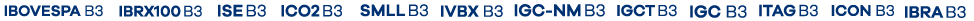• -
-
-
• -
-
-
• -
-``` (function(i, s, o, g, r, a, m) { i['GoogleAnalyticsObject'] = r; i[r] = i[r] || function() { (i[r].q = i[r].q || []).push(arguments) }, i[r].l = 1 * new Date(); a = s.createElement(o), m = s.getElementsByTagName(o); a.async = 1; a.src = g; m.parentNode.insertBefore(a, m) })(window, document, 'script', 'https://www.google-analytics.com/analytics.js', 'ga'); ga('create', 'UA-12227084-31', 'auto'); ga('send', 'pageview'); ```